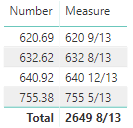2 views
in BI

I'm stumped on a seemingly simple format issue for Power BI. I'm trying to format the remainder of a number into a custom fraction (13ths)

For example 755.38 should show as 755 5/13In Excel, I use the custom fraction formula "# #/13"

In DAX I'm using the FORMAT function with the format as "#. #/13".This just takes the remainder and displays it with a "/13" on the end. it does not divide the remainder correctly.

Example 755.38 is showing as 755. 4/13 when the correct calculation is 755 5/13

by (47.2k points)
• you can put the pieces together yourself. For example,

Measure = VAR Num = SUM(Table1[Number]) VAR Frac = ROUND(13*(Num - INT(Num)), 0) RETURN FORMAT(INT(Num), "0 ") & Frac & "/13"